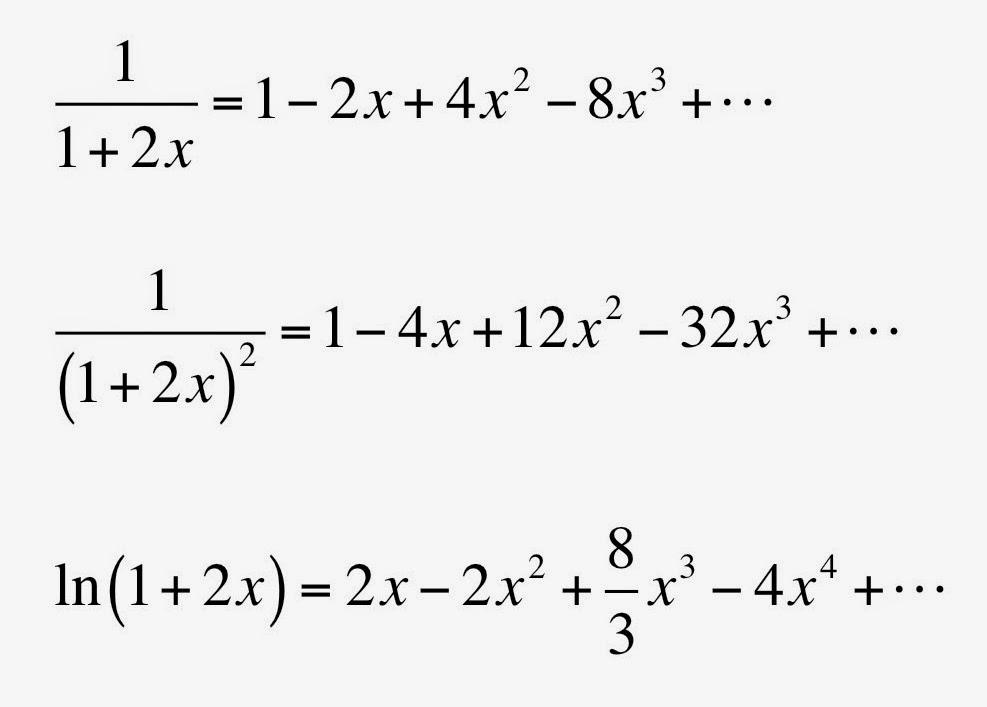Friday, February 20, 2015

The Ugliest Equation

The 4th edition of Intermediate Physics for Medicine and Biology contains thousands of equations. One of them, Equation 15.22, gives the cross section for energy transferred to electrons during Compton scattering, as a function of photon energy (x = hν/mc2). Of all the equations in the book, it is the ugliest.Eq. 15.22 in IPMB; the ugliest equation.

Russ Hobbie and I write
Equation 15.22 is a rather nasty equation to evaluate, particularly at low energies, because many of the terms nearly cancel.
Examining the behavior of the expression in brackets at small x should be easy: just take its Taylor’s series (to review Taylor’s series, see Appendix D of IPMB). In order to get the correct answer, however, you need to keep not two terms in the expansion, or three, but four! The Taylor’s series you need areThree Taylor's series needed to analyze Eq. 15.22.

Oddly, the expansion for the fourth term 4x2/3(1+2x)3 doesn’t even need one term in its expansion; it is small and doesn’t contribute to the limiting behavior. Plug these all in, and you find that the terms in x−2, x−1, and x0 all vanish. The first nonzero term is linear: 4x/3.

Out of curiosity, I evaluated each of the five terms in the expression using x = 0.01. I got

20001.9608 - 0.9900 + 9900.0192 - 0.0001 - 29900.9771  =  0.0128

The terms really do “nearly cancel.”